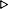ToC DocOverview CGDoc RelNotes Index PermutedIndex
Allegro CLANSI Common Lisp22 Printer22.4 Dictionary of Printer

 22.4.22 *print-level*, *print-length* Variable

Value Type:
a non-negative integer, or nil.

Initial Value:
nil.

Description:
*print-level* controls how many levels deep a nested object will print. If it is false, then no control is exercised. Otherwise, it is an integer indicating the maximum level to be printed. An object to be printed is at level 0; its components (as of a list or vector) are at level 1; and so on. If an object to be recursively printed has components and is at a level equal to or greater than the value of *print-level*, then the object is printed as "#".

*print-length* controls how many elements at a given level are printed. If it is false, there is no limit to the number of components printed. Otherwise, it is an integer indicating the maximum number of elements of an object to be printed. If exceeded, the printer will print "..." in place of the other elements. In the case of a dotted list, if the list contains exactly as many elements as the value of *print-length*, the terminating atom is printed rather than printing "..."

*print-level* and *print-length* affect the printing of an any object printed with a list-like syntax. They do not affect the printing of symbols, strings, and bit vectors.

Examples:
``` (setq a '(1 (2 (3 (4 (5 (6)))))))(1 (2 (3 (4 (5 (6))))))
(dotimes (i 8)

(let ((*print-level* i))
(format t "~&~D -- ~S~%" i a)))0 -- #1 -- (1 #)2 -- (1 (2 #))3 -- (1 (2 (3 #)))4 -- (1 (2 (3 (4 #))))5 -- (1 (2 (3 (4 (5 #)))))6 -- (1 (2 (3 (4 (5 (6))))))7 -- (1 (2 (3 (4 (5 (6))))))NIL

(setq a '(1 2 3 4 5 6))(1 2 3 4 5 6)
(dotimes (i 7)
(let ((*print-length* i))
(format t "~&~D -- ~S~%" i a)))0 -- (...)1 -- (1 ...)2 -- (1 2 ...)3 -- (1 2 3 ...)4 -- (1 2 3 4 ...)5 -- (1 2 3 4 5 6)6 -- (1 2 3 4 5 6)NIL

(dolist (level-length '((0 1) (1 1) (1 2) (1 3) (1 4)
(2 1) (2 2) (2 3) (3 2) (3 3) (3 4)))
(let ((*print-level*  (first  level-length))
(*print-length* (second level-length)))
(format t "~&~D ~D -- ~S~%"
*print-level* *print-length*
'(if (member x y) (+ (car x) 3) '(foo . #(a b c d "Baz"))))))0 1 -- #1 1 -- (IF ...)1 2 -- (IF # ...)1 3 -- (IF # # ...)1 4 -- (IF # # #)2 1 -- (IF ...)2 2 -- (IF (MEMBER X ...) ...)2 3 -- (IF (MEMBER X Y) (+ # 3) ...)3 2 -- (IF (MEMBER X ...) ...)3 3 -- (IF (MEMBER X Y) (+ (CAR X) 3) ...)3 4 -- (IF (MEMBER X Y) (+ (CAR X) 3) '(FOO . #(A B C D ...)))NIL
```